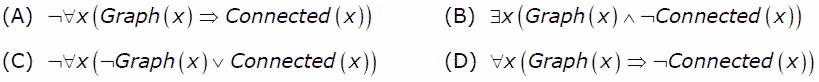# GATE | GATE-CS-2007 | Question 85

• Difficulty Level : Easy
• Last Updated : 28 Jun, 2021

Let Graph(x) be a predicate which denotes that x is a graph. Let Connected(x) be a predicate which denotes that x is connected. Which of the following first order logic sentences DOES NOT represent the statement: “Not every graph is connected”?(A) A
(B) B
(C) C
(D) D